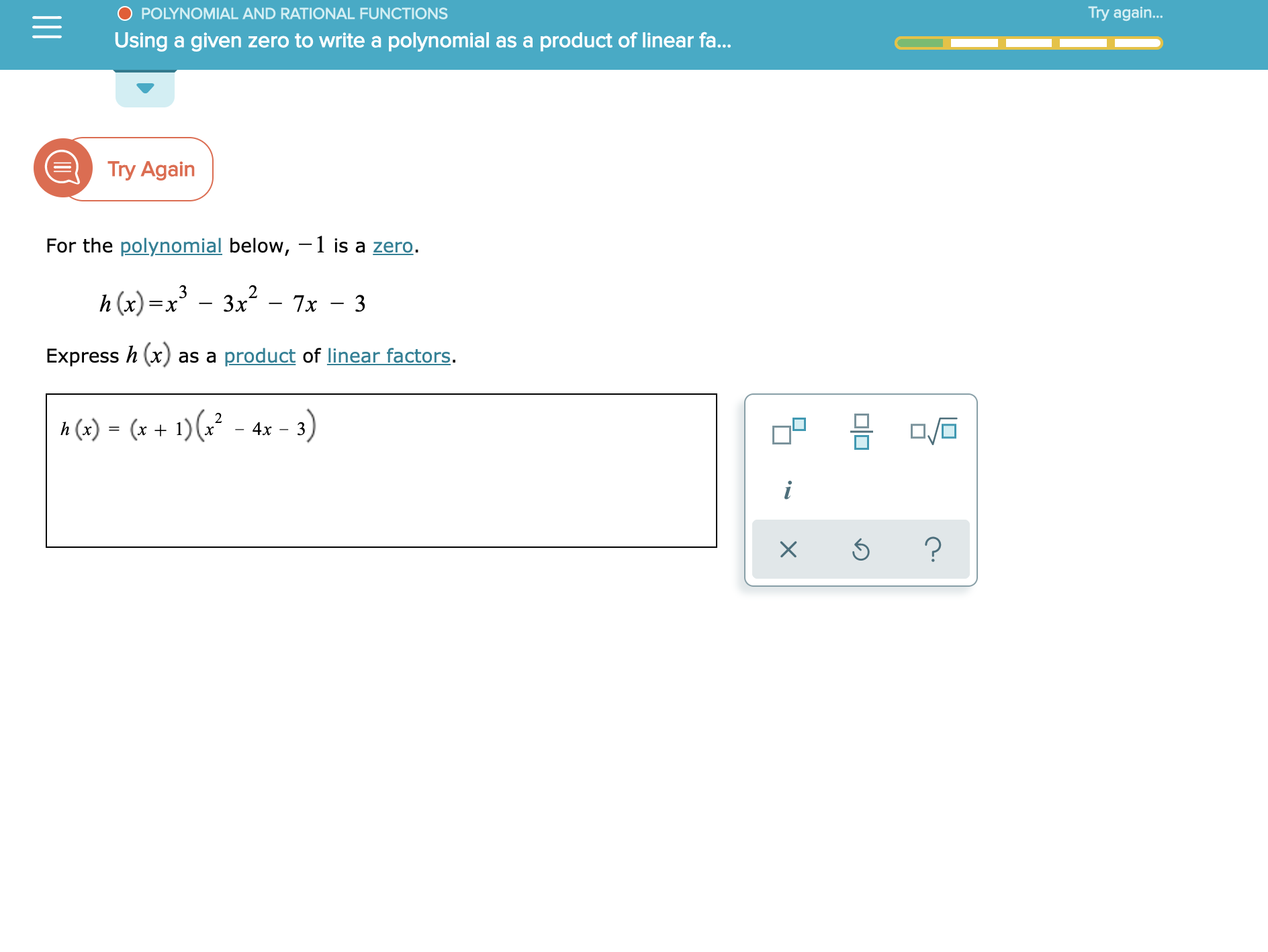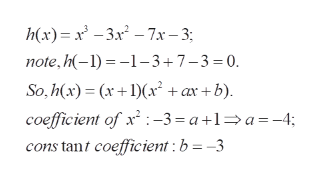# Try again...POLYNOMIAL AND RATIONAL FUNCTIONSUsing a given zero to write a polynomial as a product of linear fa...еTry AgainFor the polynomial below, -1 is a zero.h(x)x3Зx27х — 3Express h (x)as a product of linear factors.3)2h(x) (x 1)(4xi?X미□

Question
1 views

See attachmenthelp_outlineImage TranscriptioncloseTry again... POLYNOMIAL AND RATIONAL FUNCTIONS Using a given zero to write a polynomial as a product of linear fa... е Try Again For the polynomial below, -1 is a zero. h(x)x3 Зx2 7х — 3 Express h (x) as a product of linear factors. 3) 2 h(x) (x 1)( 4x i ? X 미□ fullscreen
check_circle

Step 1

To factorize h(x) completely using the given information

Step 2

As h(-1) =0, it follows that (x+1) divides h(x). Find the remaining quadratic factor , either by long division or by comparing coefficients

Step 3help_outlineImage Transcriptioncloseh(x) x-37x-3 note,h(-1)1-3+7-3 0. So,h(x)xx +ax+b) coefficient of x:-3 = a+1a-4; cons tant coefficient: b=-3 fullscreen

### Want to see the full answer?

See Solution

#### Want to see this answer and more?

Solutions are written by subject experts who are available 24/7. Questions are typically answered within 1 hour.*

See Solution
*Response times may vary by subject and question.
Tagged in

### Calculus